﻿ 基于残差自回归模型的北京市92#汽油价格的分析与预测 Analysis and Prediction of 92# Gasoline Price in Beijing Based on Residual Autoregressive Model

Vol. 08  No. 11 ( 2019 ), Article ID: 32996 , 9 pages
10.12677/ASS.2019.811257

Analysis and Prediction of 92# Gasoline Price in Beijing Based on Residual Autoregressive Model

Xiaogang Li

Yunnan University of Finance and Economics, Kunming Yunnan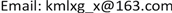Received: Oct. 28th, 2019; accepted: Nov. 11th, 2019; published: Nov. 18th, 2019ABSTRACT

Oil price is related to the national economy and people’s livelihood. This paper selects the price data of 92# gasoline for price adjustment date from January 2013 to November 2018 in Beijing. The residual autoregression model is used to investigate its fluctuation trend, extract its internal change rule and make prediction, which can provide reference for the government to adjust and control price, enterprises to make production decisions and people to maintain normal life and production order.

Keywords:Gasoline Price, Residual Autoregressive Model, ARCH Model1. 研究背景

2. 文献综述

3. 理论模型

3.1. 残差自回归模型

$\left\{\begin{array}{l}{x}_{t}={T}_{t}+{\epsilon }_{t}\\ {\epsilon }_{t}={\phi }_{1}{\epsilon }_{t-1}+\cdots +{\phi }_{p}{\epsilon }_{t-p}+{a}_{t}\end{array}$

$E\left({a}_{t}\right)=0,Var\left({a}_{t}\right)={\sigma }^{2},Cov\left({a}_{t},{a}_{t-i}\right)=0,\text{ }\forall i\ge 1$

3.1.1. 确定性模型拟合

${T}_{t}={\beta }_{0}+{\beta }_{1}\cdot t+\cdots +{\beta }_{k}\cdot {t}^{k}$，或 ${T}_{t}={\beta }_{0}+{\beta }_{1}\cdot {x}_{t-1}+\cdots +{\beta }_{k}\cdot {x}_{t-k}$

3.1.2. 残差自相关性检验及拟合

$E\left({\epsilon }_{t}{\epsilon }_{t-j}\right)\ne 0,\text{ }\exists j\ge 1$

3.2. 集群效应与ARCH模型

$\left\{\begin{array}{l}{x}_{t}=f\left(t,{x}_{t-1},{x}_{t-2},\cdots \right)+{\epsilon }_{t}\\ {\epsilon }_{t}=\sqrt{{h}_{t}}{e}_{t}\\ {h}_{t}=\omega +\underset{j=1}{\overset{q}{\sum }}{\lambda }_{j}{\epsilon }_{t-j}^{2}\end{array}$

$LM\left(q\right)=\frac{\left(SST-SSE\right)/q}{SSE/\left(T-2q-1\right)}$

4. 数据来源和处理

5. 实证分析

5.1. 残差自回归模型

$\left\{\begin{array}{l}{x}_{t}={T}_{t}+{\epsilon }_{t}，\text{ }t=1,2,3,\cdots \\ {\epsilon }_{t}={\phi }_{1}{\epsilon }_{t-1}+\cdots +{\phi }_{p}{\epsilon }_{t-p}+{a}_{t}\end{array}$

$E\left({a}_{t}\right)=0,Var\left({a}_{t}\right)={\sigma }^{2},Cov\left({a}_{t},{a}_{t-i}\right)=0,\text{ }\forall i\ge 1$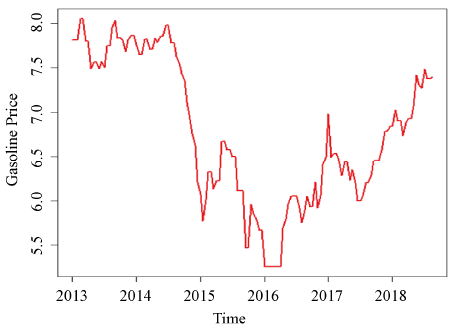Figure 1. Sequence diagram of 92# gasoline price from 2013 to 2018 (August) in Beijing

5.1.1. 确定性模型拟合

(1) 时间t的幂函数为变量：

${T}_{t}={\beta }_{0}+{\beta }_{1}\cdot t+{\beta }_{2}\cdot {t}^{2}+{\beta }_{3}\cdot {t}^{3}，\text{ }t=1,2,3,\cdots$

${x}_{t}=7.958-0.0007611{t}^{2}+0.000005572{t}^{3}+{\epsilon }_{t}\begin{array}{cc},& {\epsilon }_{t}~N\left(0,{0.3895}^{2}\right)\end{array}$Table 1. Fitting the cubic function regression model about t

(2) 1阶延迟 ${x}_{t-1}$ 为变量：

${T}_{t}={\beta }_{0}+{\beta }_{1}\cdot {x}_{t-1}$

${x}_{t}=0.13576+0.98037{x}_{t\text{-}1}+{\epsilon }_{t}\begin{array}{cc},& {\epsilon }_{t}~N\left(0,{0.1769}^{2}\right)\end{array}$Table 2. Fitting autoregressive model for delay variable

5.1.2. 残差自相关性检验及拟合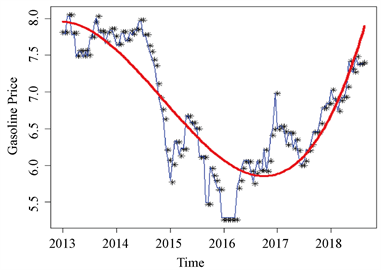Figure 2. The fitting effect of two trend-fitting models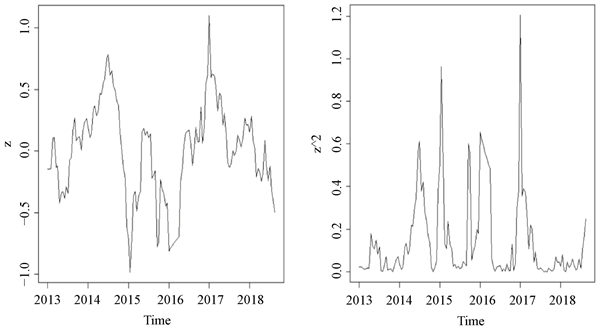Figure 3. Residual and its square after the first trend effectTable 3. DW-test on correlation of residual sequence

${\epsilon }_{t}=-0.8969{\epsilon }_{t-1}+{e}_{t}\begin{array}{cc},& {e}_{t}~N\left(0,0.02886\right)\end{array}$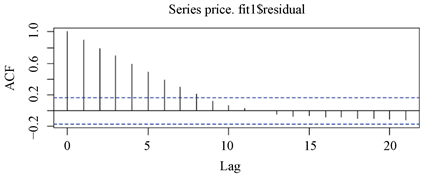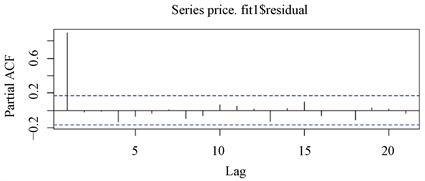Figure 4. Autocorrelation and partial autocorrelation of residual sequenceTable 4. Fitting the AR (1) model to the residual sequence

$\left\{\begin{array}{l}{x}_{t}=7.958-0.0007611{t}^{2}+0.000005572{t}^{3}+{\epsilon }_{t}\\ {\epsilon }_{t}=-0.8969{\epsilon }_{t-1}+{e}_{t}\begin{array}{cc},& {e}_{t}~N\left(0,0.02867\right)\end{array}\end{array}$Table 6. Table of predicted values, true values and relative errors

5.2. 集群效应与ARCH模型

$E\left({\epsilon }_{t}^{2}|{\epsilon }_{t-1}\right)=0.022557+0.939331{\epsilon }_{t-1}^{2}$Table 7. ARCH (1) model for residual square sequence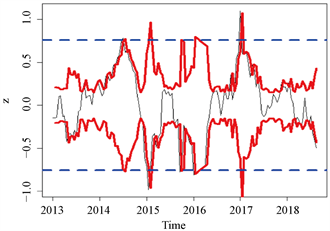Figure 5. Confidence interval comparison of conditional heteroscedasticity and homogeneity of variance

6. 结论

Analysis and Prediction of 92# Gasoline Price in Beijing Based on Residual Autoregressive Model[J]. 社会科学前沿, 2019, 08(11): 1887-1895. https://doi.org/10.12677/ASS.2019.811257

1. 1. 邢孟冉. 国际原油价格波动对我国物价影响的传导机制研究[D]: [硕士学位论文]. 济南: 山东大学国际商务, 2018.

2. 2. 晁江锋. 国际油价波动对我国宏观经济影响的研究——基于企业生产和居民消费视角的分析[J]. 价格理论与实践, 2018(3): 99-102.

3. 3. 李登伟, 郑显林, 杨剑. 油价波动下油田企业的可持续发展能力研究[J]. 北京石油管理干部学院学报, 2018, 25(4): 52-56.

4. 4. 何慧, 高登蕊, 冯长焕. 残差自回归模型的研究——基于四川省人均GDP的实证分析[J]. 兰州文理学院学报(自然科学版), 2014, 28(4): 30-32.

5. 5. 王丙参, 魏艳华, 吴欣蔓. 基于残差自回归模型的兰州市财政收入预测与决策[J]. 天水师范学院学报, 2015, 35(2): 8-11.

6. 6. 宋炜晔, 刘志媛, 王飞. 残差自回归模型在旅游经济当中的应用[J]. 现代商贸工业, 2018, 39(6): 23-25.

7. 7. 刘晓冬, 姜宝法. 残差自回归模型在中国人口出生率预测中的应用[J]. 中国卫生统计, 2011, 28(3): 309-311.

8. 8. 王璐, 杨书, 张强, 等. 残差自回归模型在艾滋病感染率变化趋势中的应用[J]. 预防医学情报杂志, 2013, 29(3): 175-177.

9. 9. 翟丽萍. 基于回归分析的97号汽油定价问题[J]. 青年科学(教师版), 2014, 35(4): 211-212.

10. 10. 徐凌, 黎佳卉, 李亮. ARIMA模型在国际原油价格预测中的应用[J]. 河南科学, 2013, 31(5): 692-695.

11. 11. 王燕. 时间序列分析——基于R[M]. 北京: 中国人民大学出版社, 2017: 161-168.